MIXING IT UP A BIT ... ANOTHER CIRCUIT, THIS TIME COMMENCING AT THE 2ND STATION.

We'll now begin another staggered station circuit, but this time starting at shaft "i". We therefore move from the Durrington Henge centre to commence orienteering from station "i".

DURRINGTON CENTRE TO i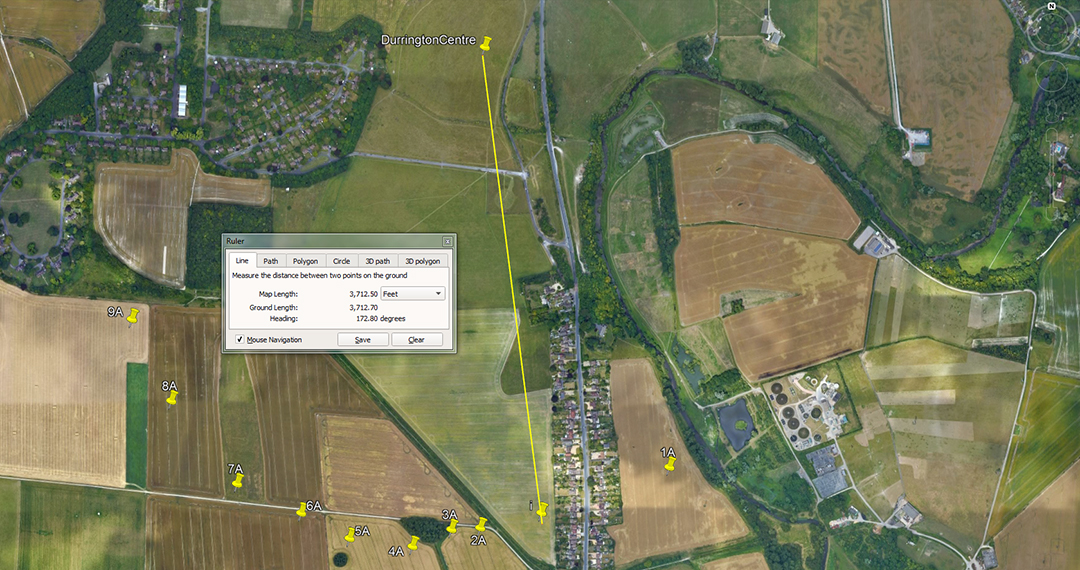The distance is 3712.5-feet from the centre of Durrington Walls Henge to station "i" and the angle is 172.8-degrees.

A mathematical progression based upon 3712.5 goes:

3712.5, 7425, 11137.5, 14850, 18562.5, 22275, 25987.5, 29700 ... 59400.

The distance relates to the Scottish mile of 5940-feet and there would be 1.6 X 3712.5 increments in 5940. Knowing as we do that the ancient Scottish mile was 1-furlong longer than the English mile and achieved 5940-feet, it's obligatory that the Scots had an Ell measurement of 37.125-inches ... which later drifted to only 37-inches (in and of itself a meaningless, orphan number ... unless read as 37.08204 ... or 6 X 6.18034-inches and based upon the PHI reciprocal of 10-inches ÷ 1.6180339 = 6.18034-inches.

A shorter form of the Ell measurement @ 37.08204-inches would only have been used for fabricating very precise capacity tubs for the marketplace or circle layouts of very accurate square footage (perhaps round houses).

i TO 3A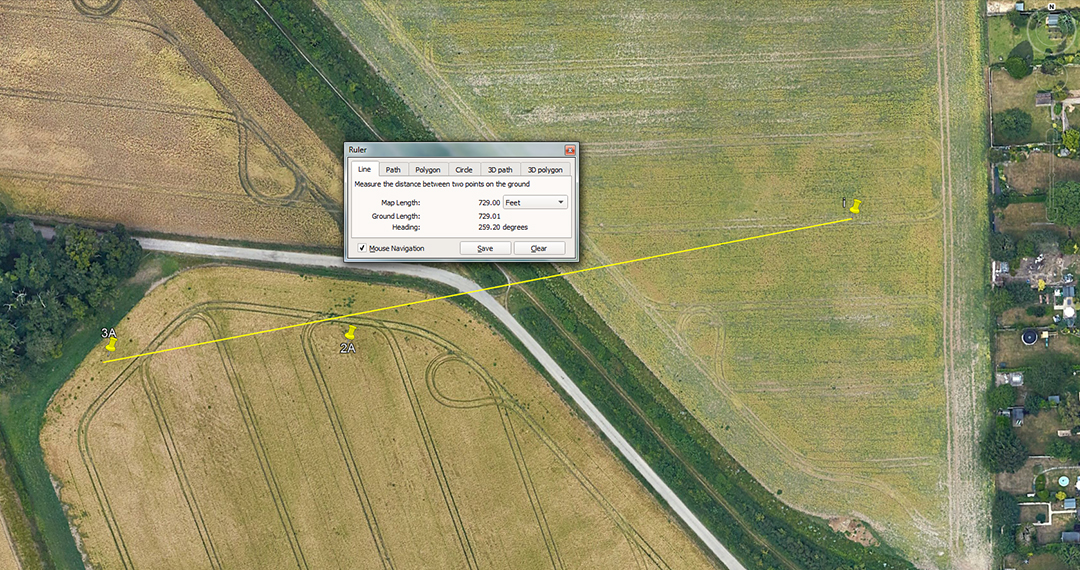The distance is 729-feet and the angle is 259.2-degrees.

A mathematical progression based upon 729 goes:

729, 1458, 2187, 2916, 3645, 4374, 5103, 5832, 6561, 7290 ... 11664 ... 25515 ... 29160 ... 46656 ... 131220000.

The sum of 7.29-inches is 1/8th of a Roman Pace (58.32-inches or 5 Roman feet of 11.664-inches). With 5000 Roman feet making a Roman mile, that equals 4860 feet of 12-inches each. With 75 Roman miles equaling 1-degree of arc (364500-feet), their reading of the equatorial circumference of the Earth was 131220000-feet or 27000 Roman miles. However, if they used the overland marching foot of 11.66666-inches, the equatorial circumference would be 131250000-feet or 25000-Greek miles of 5250-feet.

There would be 6912 Roman feet of 11.66666-inches in an Irish mile of 6720-feet.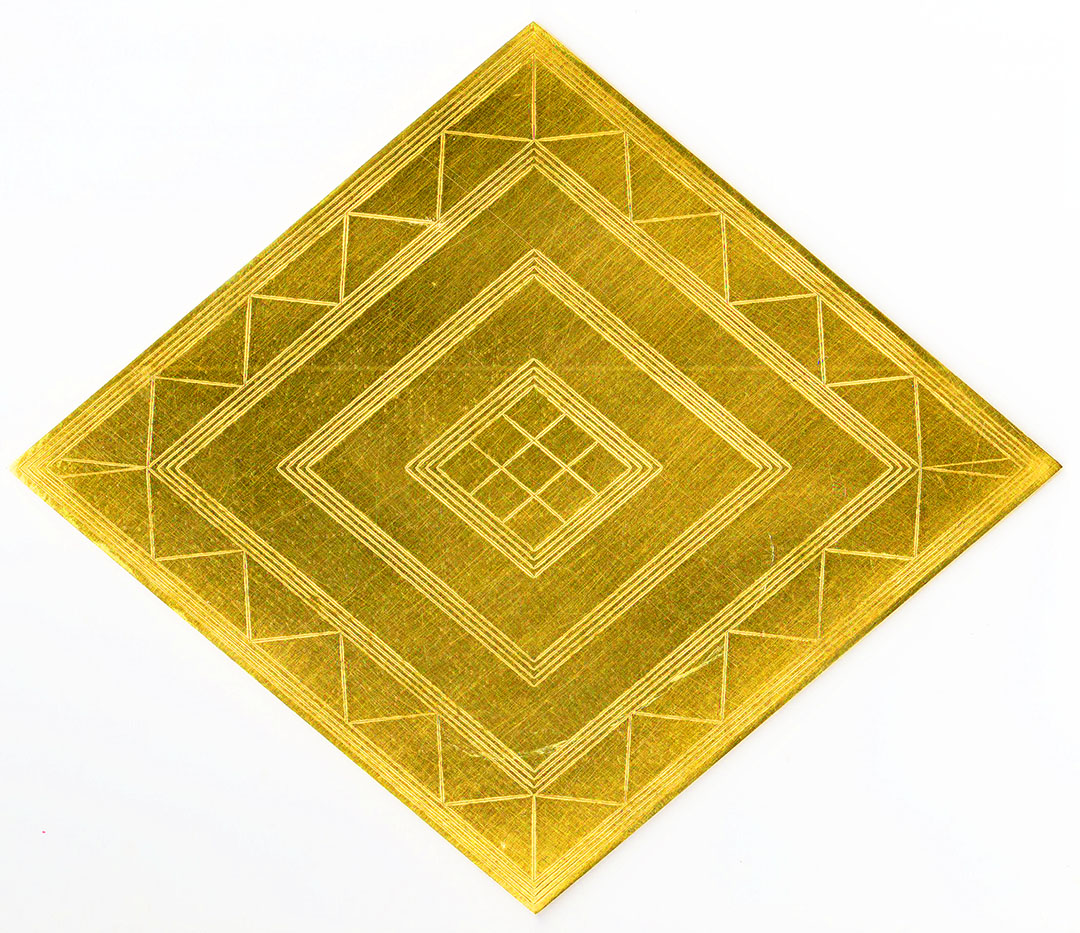The Bush Barrow Lozenge from Stonehenge is 7.29-inches in length and 6.18034-inches in width. (1/8th of a Roman foot & the PHI reciprocal based upon 10-inches ÷ PHI, respectively).

The 259.2 angle sets up the following progression:

259.2, 518.4, 777.6, 1036.8, 1296, 1555.2, 1814.4, 2073.6, 2332.8, 2592 ... 3110.4 ... 3628.8 ... 6480 ... 9072 ... 9331.2 ... 11664 ... 12441.6 ... 24883.2.

The string provides the values to describe the Earth's equatorial circumference and its divisions, as well as periods within the 25920-year duration of the Precession of the Equinoxes, etc.

Remember, Julius Caesar wrote the following concerning the Druids of his time:

'They also lecture on the stars in their motion, the magnitude of the Earth and its divisions, on natural history, on the power and government of God; and instruct the youth in these subjects' (see De Bello Gallico, VII, 15, 16.).

Isabel Hill Elder further writes, 'The students at these colleges numbered at times sixty thousand of the youth and young nobility of Britain and Gaul. Caesar comments on the fact that the Gauls sent their youth to Britain to be educated... It required twenty years to master the complete circle of Druidic knowledge. Natural philosophy, astronomy, mathematics, geometry, medicine, jurisprudence, poetry and oratory were all proposed and taught-natural philosophy and astronomy with severe exactitude.' As her sources, Elder refers to: Strabo I IV, page 197. Caesars Comm. Lib V. Sueotonius, V Calegula. E. Campion, Accounts of Ireland, pg. 18.).

3A TO 5A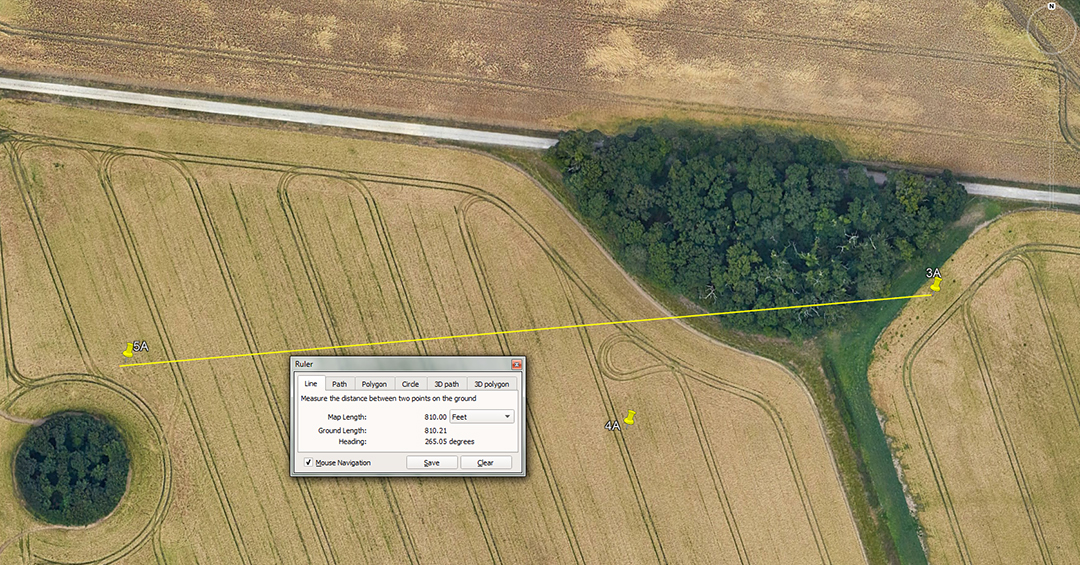The distance is 810-feet and the angle is 265.05-degrees (85.05-degrees return).

A mathematical progression based upon 810 goes:

810, 1620, 2430, 3240, 4050, 4860, 5670, 6480, 7290, 8100, 8910, 9720, 10530, 11340. 12150, 12960 ... 17010 ... 25920 ... 28350 ... 68040, 90720 ... 131220000.

These are divisions of the Earth under the Roman navigational assignment, with their mile of 4860-feet (5000 X 11.664-inches) and full equatorial circumference configured at 131220000-feet (27000 Roman miles or 25000 Greek miles. Values for the 6804-day lunar nutation cycle also occur and the Roman navigational system, like the Greek system, is based upon lunar numbers.

A mathematical progression based upon 850.5 is also lunar:

850.5, 1701, 2551.5, 3402, 4252.5, 5103, 5953.5, 6804 ... 8505 ... 13608 etc.

Again, emphasis is on divisions within the 6804-day lunar nutation cycle.

5A TO 7A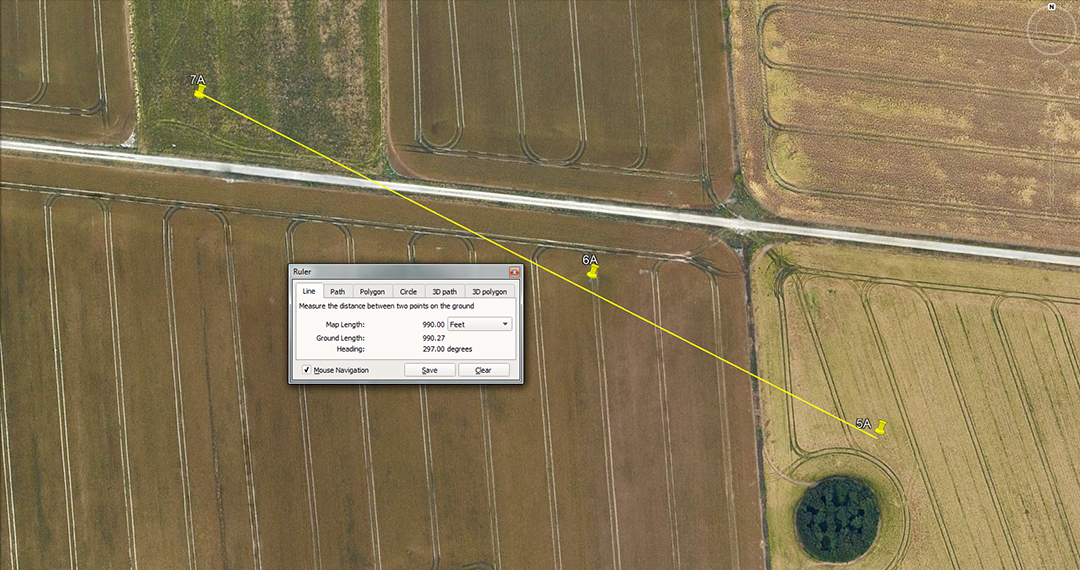The apparent length and angle codes between shaft holes was 990-feet @ 297-degrees. It's probable that the degree angle value 295.3125 could have been accommodated at more extreme diagonals between shaft holes.

A mathematical progression based upon 990 goes:

990, 1980, 2970, 3960, 4950, 5940, 6930, 7920, 8910, 9900 ... 14850 ... 17820 ... 24750 ... 65340 ... 130680000.

The sum of 990-feet is 1.5-furlongs or furrowlongs of 660-feet; 5940 is a Scottish mile which was 1-furlong greater than the English mile; 7920-miles is the diameter of the Earth; The value 178.2-feet was the diameter of "Y"-Holes at Stonehenge; 24750-miles of 5280-feet is the equatorial circumference of the Earth under the 2nd system encoded into the base dimensions of the Great Pyramid.

The string progresses through 65340 (half of 130680 to the full value, in feet, for the equatorial circumference of the Earth ... 130680000.

A mathematical progression based upon 297 goes:

297, 594, 891, 1188, 1485, 1782, 2079, 2376, 2673, 2970 ... 3564 ... 5940 ... 6534 ... 13068 ... 28512 ... 130680000.

As can be seen that this string is just another variation of the 5940 code, based upon navigating by the ancient Scottish mile. Included in this string is the value 28512, which in feet would equate to 4.8 Scottish miles. It's interesting to note that the ancient Hebrew Sepphoris Dry Volume called a Homer was 28512 cubic inches (16.5 cubic feet) and their Ephah was 2851.2 cubic inches.

Note also that the cubic capacity of a Homer volume was 16.5'cu and that 16.5-feet was 3 ancient fathoms of 5.5-feet, called a rod or perch in England. Also, 16500-feet was an ancient English league (3.125 miles of 5280 feet).

If the angle of 295.3125 could be accommodated, shaft-to-shaft, then that would relate to the lunar month of 29.53125-days (29 & 17/32nds ... accurate to within 1-minute of time).

7A TO 9A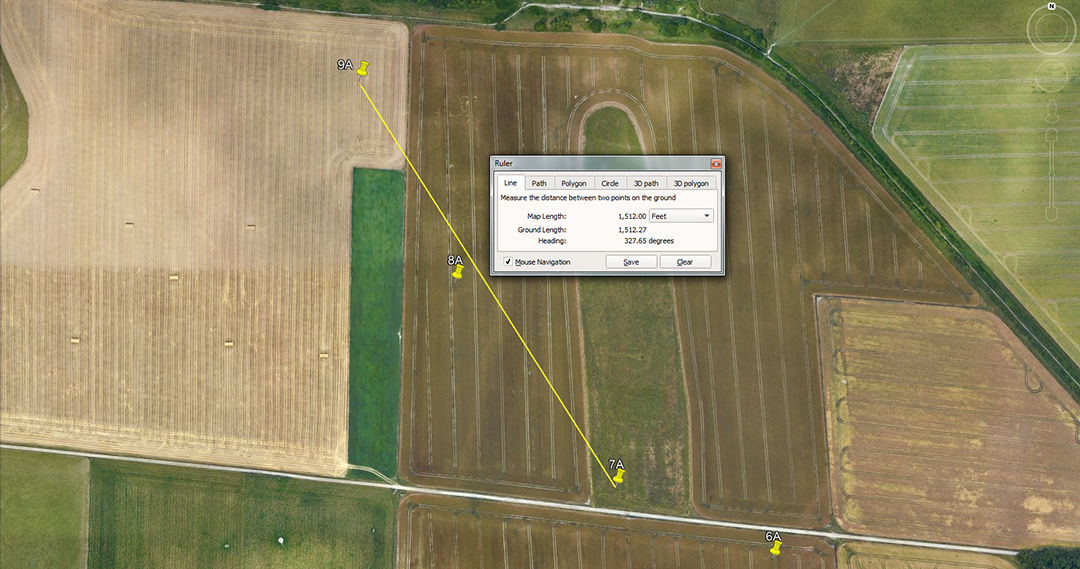The distance is 1512-feet and the return angle code is 147.65625-degrees (147 & 21/32nds ...lunar code).

A mathematical progression based upon 1512 goes:

1512, 3024, 4536, 6048, 7560, 9072, 10584, 12096, 13608, 15120 ... 22680 ... 36288 ... 362880, etc.

Running concurrently to this string would be the 2nd navigational method that used the slightly elongated reading of 756.25-feet for 1 base length of the Great Pyramid (thus 2 side readings equal 1512.5-feet).

1512.5, 3025, 4537.5, 6050, 7562.5, 9075, 10587.5, 12100, 13612.5, 15125... 22687.5 ... 36300 ... 363000, etc.

The first string provides the Great Pyramid's coded values for navigating by the mile of 5250-feet and the 2nd string by the mile of 5280-feet.

9A TO iii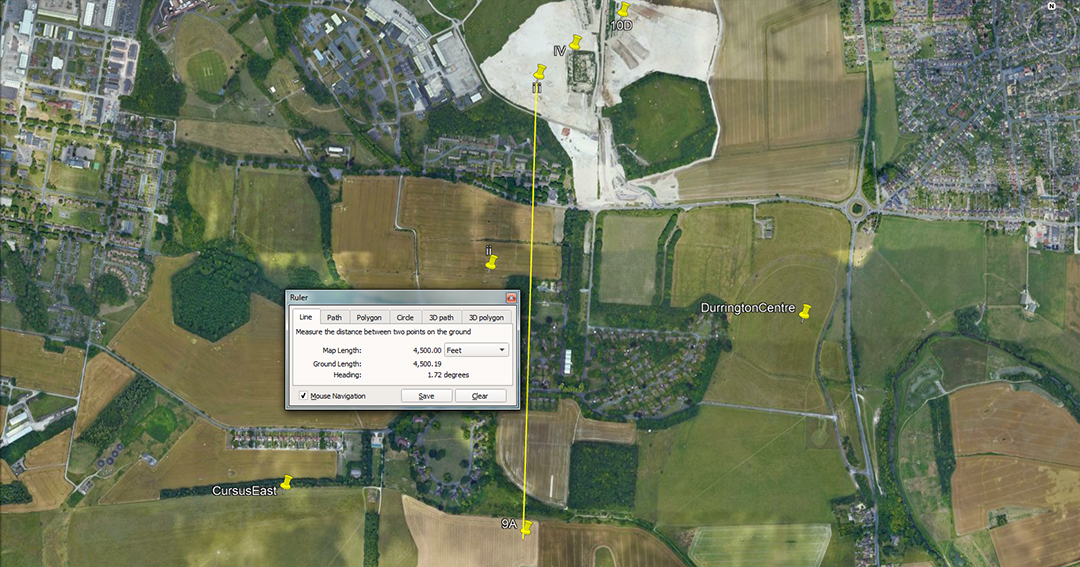The distance is 4500-feet and the angle is 1.728 degrees. The merits of the angle value, 1.75-degrees would also have featured.

A mathematical progression based upon 45 goes:

45, 90, 135, 180, 225, 270, 315, 360.

This string simply relates to primary and secondary divisions of the 360-degree compass.

The degree angle relates to the very important value 1728.

A recurring length of 1.728-feet was found by Petrie at the paving slabs of the Great Pyramid and thus lead to the identification of a cubit of 20.736-inches (1.728-feet X 12). A cubic foot is 1728-cubic inches.

iii TO 10D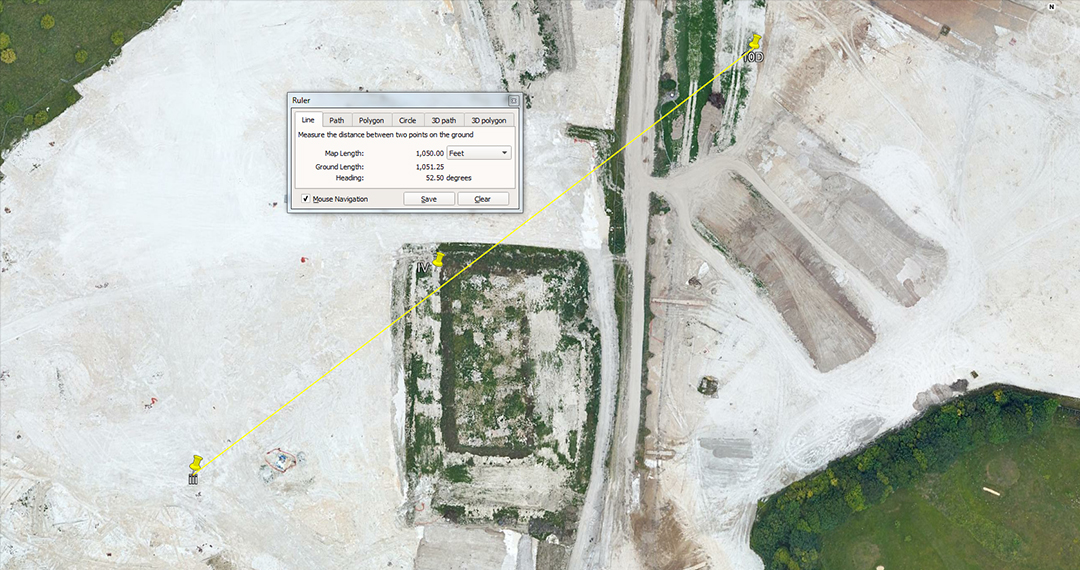The distance is 1050-feet and the angle is 52.5-degrees. The length value of 1056-feet would also have featured alongside an angle of 52.8-degrees.

The distance of 1050-feet is 100 Hebrew reeds or 1000 Greek feet. There would be 500 Hebrew reeds in a Greek mile of 5250-feet.

The distance of 1056-feet would be 1/5th of a mile of 5280-feet.

Both the distances and angles accentuate tutorials related to the respective Greek and English mile readings.

10D TO 12D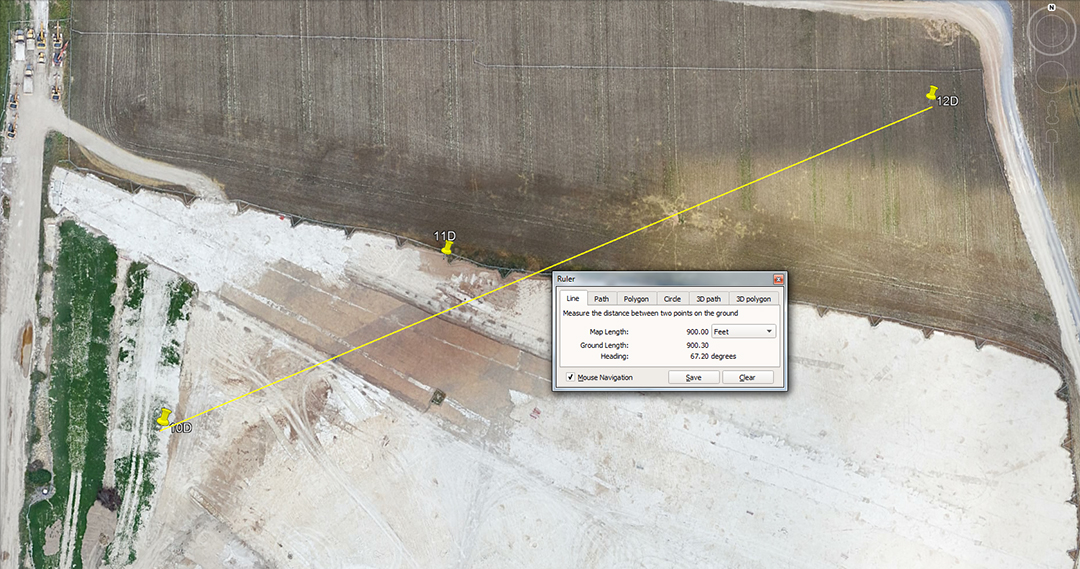The distance is 900-feet and the angle is 67.2-degrees. The opposite degree angle would also have been read to mean 247.5-degrees.

A mathematical progression based upon 90 feet goes:

90, 180, 270, 360, 450, 540, 630, 720, 810, 900, 990, 1080, 1170, 1260, 1350, 1440, 1530, 1620, 1710, 1800, 1890, 1980 ... 2160 ... 2250 ... 2520 ... 2700 ... 2880 ... 2970 ... 5940, etc.

The string provides values for the degree angle of the 4-quarters of the Earth, but also many whole number divisions, based upon 360-degree divisions within the 5940-feet environment of a Scottish mile, etc.

This infers the 5940-feet Scottish mile being used as the circumference of a circle during straight line legs of travel at sea.

Using Great Pyramid related numbers like 189-feet (1/4th of the pyramid's length) X PI @ 22/7ths = 594. Under such a system using, say, 6048-feet (1-minute of arc) for legs of travel at sea, that would convert to 19008-feet of circumference on the plotting chart or 3.2 Scottish miles of which 1/360th of the circuit was 52.8-feet per degree.

Again, using the reading for 1-minute of arc under the 5280-feet navigational encoding system of the Great Pyramid, 1-minute of arc becomes 6050-feet. This value, using PI @ 1728/550ths = 3.141818182 = 19008-feet of circumference, again providing the same reading for 1-degree of arc on the plotting chart.

If navigating by an English league of 16500-feet (3.125-miles of 5280-feet), by using PI at 1728/550ths the leg (league) converts to a circumference of 51840-feet, in which each degree of arc is 144-feet.

If navigating using the Irish mile of 6720-feet, a leg would convert to a circumference of 21120-feet using PI @ 22/7ths, generating 58.66666-feet per degree of arc on the circumference.

If navigating by the Greek stadia related value of 6300-feet (10 stadia) this could be converted using PI @ 22/7ths, generating 19800-feet of circumference in which 1-degree of arc would be 55-feet or 10 merchant navy fathoms.

The 67.2-degree angle between shafts 10D & 12D is in homage to the 6720-feet Irish mile.

The 180-degree opposed angle could be read by the tutor as representing 247.5-degrees, thus introducing the 24750-mile navigational assignment.

12D TO V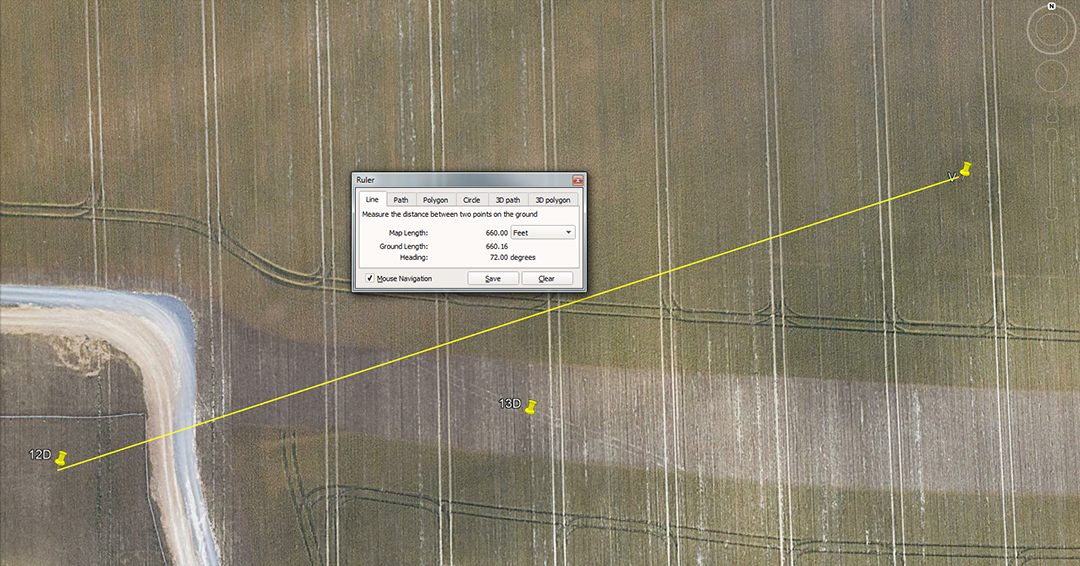The distance is 660-feet and the angle is 72-degrees or 252-degrees opposed.

This is, of course, 1-furlong or furrowlong. The mathematical progression readout is covered between stations 3A to 4A or 14D to 15D.

The progression based upon 72 is covered in the relationship between Durrington centre and shaft 1A.

The progression based upon 252 is covered between 11D to 12D.

V TO 15D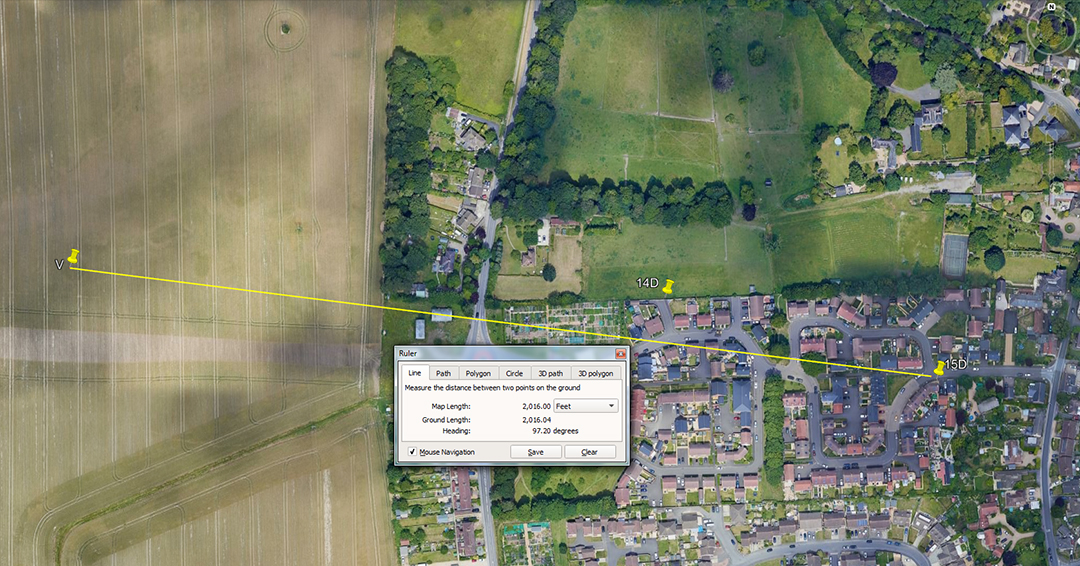The distance is 2016-feet and the angle is 97.2-degrees.

The distance of 2016-feet is, of course, 1/3rd of 1-minute of arc (6048-feet) under the Great Pyramid's navigational assignment using the mile of 5250-feet. The value could also be read a 1/3rd of 6050-feet and relate to the slightly longer mile of 5280-feet.

A mathematical progression based upon 97.2 goes:

97.2, 194.4, 291.6, 388.8, 486, 583.2, 680.4, 777.6, 874.8, 972 ... 11664 ... 1360.8 ... 1555.2 ... 2721.6 ... 3110.4 ... 6804 ... 12441.6 ... 24883.2.

These are divisions of the Earth's 24883.2-mile circumference into mostly 320th-type segments within a 360-degree environment.

V TO DURRINGTON CENTRE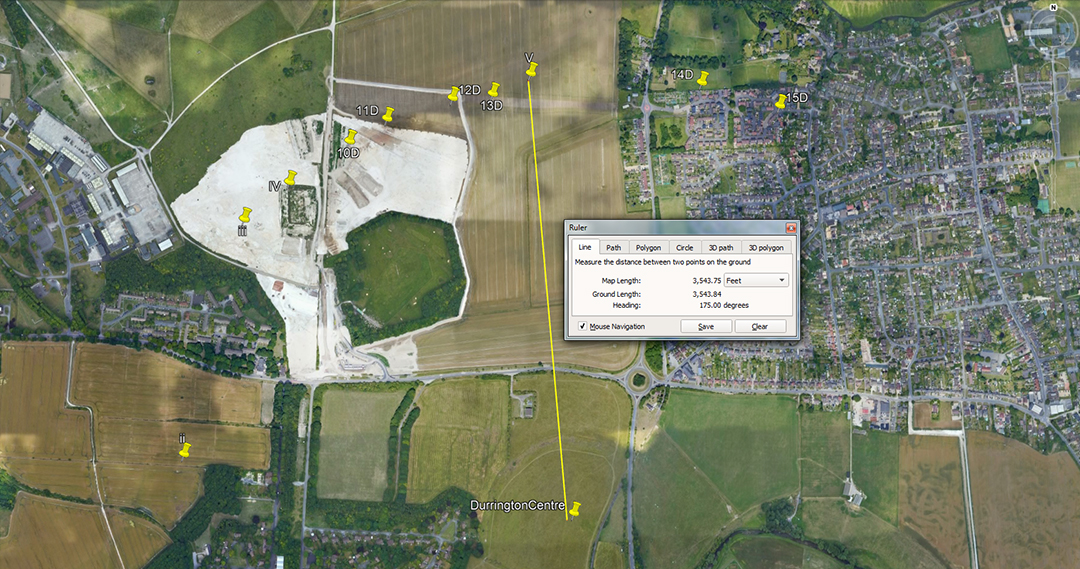The distance is 3543.75-feet and the angle is 175-degrees.

The distance is in homage to the 354.375-day duration of the lunar year.

A mathematical progression based upon the half value of 175 is adequately covered in 1A to 3A.

Continue